Shuanghe
★★

Spain,
2015-09-25 18:48
(edited by Shuanghe on 2015-09-25 19:16)

Posting: # 15471
Views: 22,048

## SAS and R (?) for variability comparison (FDA NTID Guidance) [General Sta­tis­tics]

Dear forum members,

Recent discussion on the new FDA guidance of dabigatran and rivaroxaban led me to the statistical method mentioned in the warfarin Na guidance.

It seems the method is almost the same as the one for HVDP in progesterone guidance, with difference regulatory constant and criteria of course. With the sas code example there, it's not difficult to calculate the 95% upper limit for BE evaluation.

However, there's no sas code for the variability comparison so here are my questions.

90% CI for σWTWR was expressed as {(sWT/sWR) / SQRT(F(0.05, νT, νR)), (sWT/sWR) / SQRT(F(0.95, νT, νR))}
where,
• the sWT and sWR are within-subject standard deviation for T and R, respectively;
• F(0.05, νT, νR) is the value of F-distribution with νT (numerator) and νR (denominator) degree of freedom that has probablity of 0.05;
• the similar goes for the probablity of F(0.95, νT, νR)
1. First silly question: when expressed like this I just assumed the left (blue colour) is the lower limit while the right (red colour) is the upper limit that's more important. But when I tried several data sets I always had higher value with the blue terms. Did I do anything wrong or it suppose to be so? I'm not a statistician so I don't know if F(0.05, v1, v2) is always < F(0.95, v1, v2) when v1 and v2 are kept constant.

2. From the sas code for sWR (from Dij=R1-R2 then running with PROC MIXED, output CovParms, and calculate sWR = SQRT(estimate/2)), is it correct to assume that we need to modify the code to have something like DijT = T1 - T2, then run the same procedure as for sWR to calculate sWT?

3. The unscaled sas code (method C in EMA's guideline) will output ANOVA residuals for both T and R, which were usually be used to calculate ISCV (ISCV=SQRT(EXP(residual)-1)). If so, it seems sWR and sWT can also be calculated use this approach. I tried this as well and the result is slight different from approach mentioned in point 2 (see below example). So which one should we use?

4. Since FDA request that only subject who complete all 4 periods should be included in the analysis, for obtaining F-distribution value, it seems the degree of freedom vT and vR will always be equal, which is always Ntotal - 2 for full replicate. Right?

5. I tried with EMA's sample data set I which is full replicate. There are some subjects with incomplete periods: subject 11, 20, 24, 31, 42 and 69 complete 3 periods, subject 67 and 71 complete only 2 periods. So I removed them from the data set (69 subjects left). The results are:
• sWR = 0.45168
• sWT = 0.34444
• 90% CI lower = 0.62286
• 90% CI upper = 0.93363

6. If I use unscaled method as mentioned in point 3, then the results are:
• residual R = 0.2097; sWR = SQRT(residual) = 0.45795
• residual T = 0.1202; sWT = SQRT(residual) = 0.34677
• 90% CI lower = 0.61848
• 90% CI upper = 0.92708

7. Why different? And, though the difference is small but in borderline case, one might be > 2.5 while the other might be < 2.5. So it's better to be clear which one we should approach. I prefer the former one.

I put data set here. To be more precise I didn't use the last colum logpk. instead, I calculate LOG(pk) in the program.

Could anyone check it and please let me know if there is anything wrong here? Thanks.
What I did is add a line to calculate mean difference between Test
 /* Iij and Dij. data has previously sorted by seq and subj */ DATA &pk._sabe;   MERGE &pk._t1 &pk._t2 &pk._r1 &pk._r2;   BY seq subj;     &pk._difftr = 0.5 * (&pk._t1 + &pk._t2 - &pk._r1 - &pk._r2);     &pk._diffr = &pk._r1 - &pk._r2;  /* <-- for s2wr as in the guidance sas example*/     &pk._difft = &pk._t1 - &pk._t2;  /* <-- new line for s2wt later */ RUN;

and then run an additional PROC MIXED

/* Intermediate analysis of Dij(T), the mean diff between T1 and T2. */ PROC MIXED DATA = &pk._sabe;     CLASS seq;     MODEL &pk._difft = seq / DDFM = SATTERTH;     ESTIMATE "&pk" INTERCEPT 1 seq 0.5 0.5 / E CL ALPHA = 0.1;     ODS OUTPUT COVPARMS = &pk._difft_out1;     ODS OUTPUT ESTIMATES = &pk._difft_out2;     ODS OUTPUT NOBS = &pk._difft_out3;     ODS OUTPUT TESTS3 = &pk._difft_out4; TITLE6 "Scaled Average BE, Intermediate Analysis of Dij(T), PROC MIXED"; TITLE7 " ";  RUN; DATA &pk._difft_out1;     SET &pk._difft_out1;     s2wt = ESTIMATE / 2;     KEEP s2wt; RUN;

and then calculate 90% interval for variability comparison

DATA &pk._final;     MERGE &pk._difftr_out &pk._difft_out1 &pk._difft_out2 &pk._diffr_out1 &pk._diffr_out2;     theta = (LOG(1 / 0.9) / 0.1)**2;     y = -theta * s2wr;     boundy = y * dfr / CINV(0.95, dfr);     swr = SQRT(s2wr);     swt = SQRT(s2wt);     critbound = (x + y) + SQRT((boundx - x)**2 + (boundy - y)**2);     varlower = (swt / swr) / SQRT(FINV(0.05, dft, dfr)); /* <-- actually upper */     varupper = (swt / swr) / SQRT(FINV(0.95, dft, dfr)); /* <-- actually lower */ RUN;

Correct?

8. Based on the description of the method in the guidance, it seems the analysis can be done in R. Has anyone tried it yet? I'll give it a try and report the result later.

9. Helmut, this is for you. It seems LaTeX expression can not be used to write math equation here. Is it possible to implement it? Since there are so many members here and some times one has to write complicate formula it might be a good idea to be able to write equations in LaTeX.OK, that's for now. I wish you all a wonderful weekend!

All the best,
Shuanghe
jag009
★★★

NJ,
2015-09-25 22:42

@ Shuanghe
Posting: # 15473
Views: 19,263

## SAS and R (?) for variability comparison (FDA NTID Guidance)

Hi,

» 4. Since FDA request that only subject who complete all 4 periods should be included in the analysis, for obtaining F-distribution value, it seems the degree of freedom vT and vR will always be equal, which is always Ntotal - 2 for full replicate. Right?

Where did you see this? I don't see it in the guidance. However, if you use FDA's SAS code (ie: Progesterone) to extract the variances etc etc, you WILL see that the code only takes subjects who completed both periods for Test and Ref. Example, any subjects who finished only 1T out of 2Ts will be dropped.

Take a look at previous threads involving the Concerta guidance (I started that thread and a few joined in. Sorry I don't have time to set the link now. Need to go party).Lastly, as to why you get reverse upper and lower limit with the interval, I will try and goof around in SAS next week and see.

John

Edit: The threads John mentioned above: #13991, #14150. [Helmut]
Shuanghe
★★

Spain,
2015-09-28 17:05

@ jag009
Posting: # 15478
Views: 18,971

## SAS and R (?) for variability comparison (FDA NTID Guidance)

Hi John,

» Where did you see this? I don't see it in the guidance. However, if you use FDA's SAS code (ie: Progesterone) to extract the variances etc etc, you WILL see that the code only takes subjects who completed both periods for Test and Ref. Example, any subjects who finished only 1T out of 2Ts will be dropped.

That's what I meant. By FDA's code, all subject has to complete all 4 periods. so In practice, number of subjects having T data is always equal number of to subjects having R data.

By the way, I asked FDA about possibility of modify the code to include subject with 2 R and 1 T and for "ilat", the mean difference between T and R, use the modified version:
ilat = lat1t - 0.5*(lat1r+lat2r) ,
where lat1t could be replaced by lat2t depending on period of dropout. It should be easy to implement with IF/ELSE/THEN. Buy they confirmed 1 and a half years later that subjects should have data of all 4 periods and reject the suggestion.

» Take a look at previous threads involving the Concerta guidance (I started that thread and a few joined in. Sorry I don't have time to set the link now. Need to go party).» Edit: The threads John mentioned above: #13991, #14150. [Helmut]

Woo, long post! I'll definitely take a look at them later. Thanks.

All the best,
Shuanghe
d_labes
★★★

Berlin, Germany,
2015-09-30 10:52

@ Shuanghe
Posting: # 15497
Views: 18,421

## Missings

Dear Shuanghe!

» ... By FDA's code, all subject has to complete all 4 periods. so In practice, number of subjects having T data is always equal number of to subjects having R data.
»
» By the way, I asked FDA about possibility of modify the code to include subject with 2 R and 1 T and for "ilat", the mean difference between T and R, use the modified version:
» ilat = lat1t - 0.5*(lat1r+lat2r) ,
» where lat1t could be replaced by lat2t depending on period of dropout. It should be easy to implement with IF/ELSE/THEN. Buy they confirmed 1 and a half years later that subjects should have data of all 4 periods and reject the suggestion.

What you describe is the part of the SAS code concerning (µT-µR)^2 of the linearized scABE criterion (ilat analysis). For the part dealing with intra-subject variability the Progesterone / warfarin guidance SAS code (dlat analysis) does not automatically drop subjects having missings under Test treatment.

What to do here? Retain subjects having 2R, but only 1T or 0T? Or also drop them as we have done in the R code in the post below?

Regards,

Detlew
Shuanghe
★★

Spain,
2015-09-30 15:34

@ d_labes
Posting: # 15502
Views: 18,353

## Missings

Dear Detlew,

» What you describe is the part of the SAS code concerning (µT-µR)^2 of the linearized scABE criterion (ilat analysis).

You are right.

» For the part dealing with intra-subject variability the Progesterone / warfarin guidance SAS code (dlat analysis) does not automatically drop subjects having missings under Test treatment.

Yes. And I tried both with some of my studies. The difference is small but in borderline case it could mean pass or fail BE

» What to do here? Retain subjects having 2R, but only 1T or 0T? Or also drop them as we have done in the R code in the post below?

In addition to the scenarios you mentioned, what about subject with 0R + 2T? Theoretically they can provide information on ISCV for T.
To make a list of what cannot be included is much easier,
• 1T or 1R
• 1T+1R
All the rest combinations can provide ISCV either for T or for R or for all.

So, for the moment I prefer using subjects with all 4 periods just to be safe (provided it was properly described in the protocol). Otherwise, situation would be more complicated.

All the best,
Shuanghe
Helmut
★★★Vienna, Austria,
2015-09-27 14:23

@ Shuanghe
Posting: # 15474
Views: 19,313

## LaTeX, MathML, PNGs

Hi Shuanghe,

congratulations for mastering the nested lists in your post. I’m impressed!

» 9. It seems LaTeX expression can not be used to write math equation here.

Correct.

» Is it possible to implement it?

Theoretically yes.
• It would be possible to add GeSHi which was also used by Wikipedia. In version 2 of the forum’s scripts it turned out that the server-load can be extreme (though fine for distributed sites like WP). It lead to a work­around almost doubling the size of the MySQL-database (one table containing original posts and another one linking to rendered PNGs; example-post). I didn’t like that and decided to stay with the v1.x-branch.
• Another – desirable – option would allow to embed MathML in (X)HTML. Currently the support by browsers is limited (see here; unfortunately 11.1% of users of the forum still on IE).
• Client-side solutions (KaTeX, MathQuill, MathJaxSHJS, …) are a not so nice because some com­pa­nies’ IT-folk restrict JavaScript on user’s machines.
If you enjoy the “beauty” of formulas:⇒ DVI ⇒ Ghostscript ⇒ grayscale (4 or 8 bit) PNG with alpha-trans­parency en­abled and upload/link the file here. Example: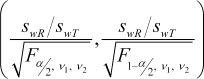Drawbacks: If images are dis­abled in the browser only the image’s attribute alt="[image]" is displayed. Ge­SHi would come up with alt="\left ( \frac{s_{wR} / s_{wT} } {\sqrt{F_{\alpha/2}, \quad {\nu_1}, \quad {\nu_2}}}, \frac{s_{wR} / s_{wT} } {\sqrt{F_{1-\alpha/2}, \quad {\nu_1}, \quad {\nu_2}}} \right )". The former is non-informative and the latter confusing to anybody not familiar with the syntax of AMS.

BTW, simple formulas in the forum can be constructed by means of UTF-8 characters and BB-Codes, e.g.,

CVw = √σ²w − 1

» Since there are so many members here …

Don’t overestimate that. This year so far only 122 of them were active (at least one post). The forum is a rather exclusive club; ten nerds (0.1%) are guilty for 55% of all posts.

» … and some times one has to write complicate formula it might be a good idea to be able to write equations in LaTeX.

I’m afraid users in the forum being knowledgable ofare in the minority… BTW, I once wrote a manu­script in MiKTEX according to the publisher’s templates only to learn that they required bloody M$-Word in the mean­time. Although I used MathType don’t ask me how the paper looked at the end.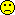Edit: With the MathJax library (installed June 2019): $$\left(\frac{s_{wR}/s_{wT}} {\sqrt{F_{\alpha/2},\quad{\nu_1},\quad{\nu_2}}},\frac{s_{wR}/s_{wT} }{\sqrt{F_{1-\alpha/2},\quad{\nu_1},\quad{\nu_2}}}\right)$$ $$C{V_w}=\sqrt{{e^{\sigma _w^2}}-1}$$ Cheers, Helmut SchützThe quality of responses received is directly proportional to the quality of the question asked. 🚮 Science Quotes Shuanghe ★★ Spain, 2015-09-28 17:32 @ Helmut Posting: # 15479 Views: 18,859 ## OT: LaTeX, MathML, PNGs Hi Helmut, » congratulations for mastering the nested lists in your post. I’m impressed!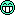» Theoretically yes. • ... • Another... • Client-side solutions... That's way too more complicated than I thought. I'll just upload png if it's necessary in future. » I’m afraid users in the forum being knowledgable ofare in the minority… BTW, I once wrote a manu­script in MiKTEX according to the publisher’s templates only to learn that they required bloody M$-Word in the mean­time.

If they need $Word, why bother provide LaTeX template?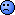No, the correct questions is since they have LaTeX template, why bother requiring$Word at all? TeX produce document with much better quality.» Although I used MathType don’t ask me how the paper looked at the end.By the way, I have TeX Live installed and use TeXStudio as editor. It works great. I once send 12 pages pdf answering a regulatory question about multivariate statistical distance method for dissolution comparison with tufte-handout class with many side notes illustrations. Very "professional printout"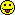TeX rocks!

All the best,
Shuanghe
Helmut
★★★Vienna, Austria,
2015-09-28 17:46

@ Shuanghe
Posting: # 15481
Views: 18,873

## OT: LaTeX, MathML, PNGs

Hi Shuanghe,

» […] I'll just upload png if it's necessary in future.It is possible to get a PNG from one of Google’s tools. Paste the TEX-code after https://chart.apis.google.com/chart?cht=tx&chl=
Open this link in a new tab:

https://chart.apis.google.com/chart?cht=tx&chl=\left ( \frac{s_{wR} / s_{wT} } {\sqrt{F_{\alpha/2}, \quad {\nu_1}, \quad {\nu_2}}}, \frac{s_{wR} / s_{wT} } {\sqrt{F_{1-\alpha/2}, \quad {\nu_1}, \quad {\nu_2}}} \right )

Not perfect, but OK. It’s a 24 bit PNG.

» » […] I once wrote a manu­script in MiKTEX according to the publisher’s templates only to learn that they required bloody M$-Word in the mean­time. » If they need$Word, why bother provide LaTeX template?» No, the correct questions is since they have LaTeX template, why bother requiring $Word at all? You missed the phrase “in the meantime”. Of course they removed the TEX-stuff from their website and provided M$-DOTs instead. Why? Compare the user-base of M$-Word with the one of all flavors of TEX » TeX produce document with much better quality.Absolutely. » […] I have TeX Live installed and use TeXStudio as editor. I will give it a try! Cheers, Helmut SchützThe quality of responses received is directly proportional to the quality of the question asked. 🚮 Science Quotes d_labes ★★★ Berlin, Germany, 2015-09-28 09:45 @ Shuanghe Posting: # 15475 Views: 18,980 ## Quantiles of the F-distribution Dear Shuanghe, » However, there's no sas code for the variability comparison ... Wow! Seems correct. Was not aware of this fact. At moment I don't have answers to all of your other questions but only to your points 1., 2. and 4. Lets start with 2. and 4, the simpler ones: » 2. From the sas code for sWR (from Dij=R1-R2 then running with PROC MIXED, output CovParms, and calculate sWR = SQRT(estimate/2)), is it correct to assume that we need to modify the code to have something like DijT = T1 - T2, then run the same procedure as for sWR to calculate sWT? Correct. » 4. Since FDA request that only subject who complete all 4 periods should be included in the analysis, for obtaining F-distribution value, it seems the degree of freedom vT and vR will always be equal, which is always Ntotal - 2 for full replicate. Right? Correct for full replicate 4-periods with 2 sequences with mentioned precondition. Now to the mysterious lower/upper (upper/lower?) CI of the variabilities (your point 1.): The answer lies hidden in the text of the Warfarin guidance. "... Fα/2,ν1,ν2 is the value of the F-distribution with ν1 (numerator) and ν2 (denominator) degrees of freedom that has probability of α/2 to its right." (Emphasis be me. Similar text for F1-α/2,ν1,ν2). Example in R speak with df1=12, df2=12, alpha/2=0.05: F1 <- qf(0.05, df1=12, df2=12, lower.tail=FALSE) gives F1 = 2.686637. This is the same as F1 <- qf(1-0.05, df1=12, df2=12, lower.tail=TRUE) Usually (and in SAS) the probability to the left is given back (integral over F-density from 0 to F). # lower.tail=TRUE is default F2 <- qf(0.05, df1=12, df2=12) gives F2 = 0.3722125. SAS: Data Finv; df1=12; df2=12; alpha=0.05; F1=Finv(1-alpha, df1, df2); F2=Finv(alpha, df1, df2); run; gives also F1 = 2.686637, F2 = 0.3722125. Hope this helps. Regards, Detlew Shuanghe ★★ Spain, 2015-09-28 17:39 @ d_labes Posting: # 15480 Views: 18,859 ## Quantiles of the F-distribution Dear Detlew, » Lets start with 2. and 4, the simpler ones: » Correct for full replicate 4-periods with 2 sequences with mentioned precondition. Thanks for the confirmation. » Now to the mysterious lower/upper (upper/lower?) CI of the variabilities (your point 1.): » The answer lies hidden in the text of the Warfarin guidance. » "... Fα/2,ν1,ν2 is the value of the F-distribution with ν1 (numerator) and ν2 (denominator) degrees of freedom that has probability of α/2 to its right." (Emphasis be me. Similar text for F1-α/2,ν1,ν2). Thanks!!! Didn't noticed that.» Hope this helps. Yes!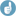All the best, Shuanghe Shuanghe ★★ Spain, 2015-09-28 18:57 @ Shuanghe Posting: # 15482 Views: 19,207 ## What's wrong with this picture? Dear all, When I said it seems the analysis is doable in R I made at least 2 mistakes. 1. I forgot the step 3 mentioned in warfarin's guidance. The 90% CI analysed by unscaled BE should be inside 80-125%. This (replicate BE), if I recall one of Detlew's earlier posts correctly, cannot be done in R at the moment, at least in the sense of "reproduce" SAS output. 2. Step 2 in guidance mentioned that 95% upper CI should be calculated as: (YT - YR)2 - θ * s2WR, where "YT and YR are means of the ln-transformed PK endpoint" for T and R respectively, which is easy to calculate (or so I thought...) Let's fotget the 90% CI since it's impossible to do so in R. I played a bit with R for calculation of the rest parameters as a way of learning R and here it is the code. What's wrong with this picture (see comments in red colour)? # read data from website, variable names: subj, per, seq, treat, pk, logpk emadata <- "https://dl.dropboxusercontent.com/u/7685360/permlink/emabe4p.csv" ema <- read.csv(file = emadata, colClasses = (c(rep("factor", 4), rep("numeric", 2)))) # remove subjects who has imcomplete periods. More elegant solution? library(dplyr) d <- filter(ema, subj != 11 & subj != 20 & subj != 24 & subj != 31 & subj != 42 & subj != 67 & subj != 69 & subj != 71) # create data sets containing only R1, R2, T1 and T2, respectively. # change variable name accordingly and drop unnecessary variables. r1 <- d %>% filter((seq == "TRTR" & per == 2) | (seq == "RTRT" & per == 1)) %>% mutate(pkr1 = logpk) %>% # rename variable select(-c(per, treat, pk, logpk)) # drop variables r2 <- d %>% filter((seq == "TRTR" & per == 4) | (seq == "RTRT" & per == 3)) %>% mutate(pkr2 = logpk) %>% select(-c(per, treat, pk, logpk)) t1 <- d %>% filter((seq == "TRTR" & per == 1) | (seq == "RTRT" & per == 2)) %>% mutate(pkt1 = logpk) %>% select(-c(per, treat, pk, logpk)) t2 <- d %>% filter((seq == "TRTR" & per == 3) | (seq == "RTRT" & per == 4)) %>% mutate(pkt2 = logpk) %>% select(-c(per, treat, pk, logpk)) # merge data sets r12 <- merge(r1, r2, by = c("seq", "subj")) t12 <- merge(t1, t2, by = c("seq", "subj")) drt <- merge(r12, t12, by = c("seq", "subj")) # calculate mean difference between T and R, diff between R1 and R2, T1 and T2 scavbe <- mutate(drt, diff_tr = 0.5 * (pkt1 + pkt2 - pkr1 - pkr2), # FDA's ilat diff_r = pkr1 - pkr2, # FDA's dlat, for swr estimation diff_t = pkt1 - pkt2) # additional variable to estimate swt # calculate mean difference by sequence --------------------- mdiff <- scavbe %>% group_by(seq) %>% summarise(size = n(), # number of subjects mdiff_tr = mean(diff_tr), mdiff_r = mean(diff_r), mdiff_t = mean(diff_t)) # calculate swr and swt. same as SAS output all <- merge(scavbe, mdiff, by = "seq") sw <- all %>% mutate(ss_r = (diff_r - mdiff_r)**2, ss_t = (diff_t - mdiff_t)**2) %>% summarise(s2wr = sum(ss_r) / (2*(n() - 2)), # formula in FDA's guidance s2wt = sum(ss_t) / (2*(n() - 2)), swr = sqrt(s2wr), swt = sqrt(s2wt)) # 95% upper CI as (mean(T) - mean(R))**2 - theta*s2wr. Finding mean T and R # Should mean value be calculated like this? It seems too simple. But if not, how? t <- filter(d, treat == "T") %>% mutate(mean_t = mean(logpk)) %>% distinct(treat) %>% select(mean_t) r <- filter(d, treat == "R") %>% mutate(mean_r = mean(logpk)) %>% distinct(treat) %>% select(mean_r) # critbound value is different from sas output. # 90% CI for sigma(T)/sigma(R) are same as sas output tr <- merge(t, r) final <- merge(sw, tr) %>% mutate(size = nrow(distinct(d, subj)), theta = (log(1/0.9)/0.10)**2, critbound = (mean_t - mean_r)**2 - theta * s2wr, ## different from SAS. cilower = swt/swr/(sqrt(qf(0.05, df1 = size - 2, df2 = size -2, lower.tail = FALSE))), ciupper = swt/swr/(sqrt(qf(0.95, df1 = size - 2, df2 = size -2, lower.tail = FALSE))))  So to summarise, I can get sWR, sWT, 90% CI for variability comparison (Thanks to Detlew), but not 95% upper limit for (YT - YR)2 - θ * s2WR. I'm sure the error comes from the mean T and R. Any thought? All the best, Shuanghe Helmut ★★★Vienna, Austria, 2015-09-29 02:22 @ Shuanghe Posting: # 15489 Views: 18,790 ## Suggestion Hi Shuanghe, » # remove subjects who has imcomplete periods. More elegant solution?» library(dplyr) Didn’t know this package. The syntax within some functions (i.e., the %>%-operator) is – well – un­con­ven­tio­nal. Code to keep only subjects completing all four periods: summary(ema[2:4]) # show structure per.4 <- summary(ema$subj) == 4 # subjs have 4 levels? incomp <- per.4[per.4 == FALSE]  # incomplete ones print(sort(names(incomp))) comp   <- per.4[per.4 == TRUE]   # complete ones print(sort(names(comp))) d      <- subset(ema, subj %in% names(comp)) print(d) summary(d[2:4]) seq1   <- as.numeric(summary(d[, 3])[names(summary(d[, 3]))]) seq2   <- as.numeric(summary(d[, 3])[names(summary(d[, 3]))]) if(seq1 == seq2) {  txt <- "Balanced: "  } else {  txt <- "Unbalanced: " } cat(paste0(txt, names(summary(d[, 3])), "=", seq1, ", ",  names(summary(d[, 3])), "=", seq2, "\n"))

Lines in red can be dropped (checking results only).

Which subjects are incomplete? print(sort(names(incomp))) gives

 "11" "20" "24" "31" "42" "67" "69" "71"summary(d[2:4]) gives

 per      seq      treat  1:69   RTRT:144   R:138  2:69   TRTR:132   T:138  3:69  4:69

and the goody

Unbalanced: RTRT=144, TRTR=132

The code works for fancy non-numeric subject-IDs and other sequence-codings as well. Try

ema$subj <- as.factor(paste0("Sub #", ema$subj)) levels(ema$seq)[levels(ema$seq)=="RTRT"]  <- 1 levels(ema$seq)[levels(ema$seq)=="TRTR"]  <- 2 levels(ema$treat)[levels(ema$treat)=="T"] <- "A" levels(ema$treat)[levels(ema$treat)=="R"] <- "B"

and run the code. I got for print(sort(names(incomp)))

 "Sub #11" "Sub #20" "Sub #24" "Sub #31" "Sub #42" "Sub #67" "Sub #69"  "Sub #71

and finally for summary(d[2:4])

 per    seq     treat  1:69   1:144   B:138  2:69   2:132   A:138  3:69         4:69

Same as above (apart from differing sequences and treatments).Edit: Once I saw Detlew’s code below, I can only say about my snippet: Forget it!

Cheers,
Helmut SchützThe quality of responses received is directly proportional to the quality of the question asked. 🚮
Science Quotes
Shuanghe
★★

Spain,
2015-09-29 18:44

@ Helmut
Posting: # 15494
Views: 18,551

## Suggestion

Hi Helmut,

» Didn’t know this package. The syntax within some functions (i.e., the %>%-operator) is – well – un­con­ven­tio­nal.

The package was widely talked about recently on various forums and supposedly very powerful. Well, for those who mastered it I guess, which I'm not obviously.» Edit: Once I saw Detlew’s code below, I can only say about my snippet: Forget it!

For beginner like me, definitely I can learn from both.All the best,
Shuanghe
d_labes
★★★

Berlin, Germany,
2015-09-30 08:44

@ Shuanghe
Posting: # 15496
Views: 18,570

Dear Shuanghe, dear Helmut,

» » Didn’t know this package. The syntax within some functions (i.e., the %>%-operator) is – well – un­con­ven­tio­nal.
»
» The package was widely talked about recently on various forums and supposedly very powerful. Well, for those who mastered it I guess, which I'm not obviously.There is much hype within the R-user community around Hadley Wickham (has even an entry in Wikipedia) and the wealth of packages - named "Hadleyverse" - he has written, among them prominently plyr / dplyr.

Hadley has got headlines like "The man who revolutionized R" and has got fans all over the world.

I myself don't understand this hype. I find his packages at least in part not so easy to use or understand.
What I really love is devtools for package development (integral part of R-Studio, the IDE which no R-user/developer should miss) and his book "Advanced R".

Regards,

Detlew
d_labes
★★★

Berlin, Germany,
2015-09-29 14:26

@ Shuanghe
Posting: # 15490
Views: 19,129

## Another suggestion for a R-solution

Dear Shuanghe, dear all,

here my two cents (only base R, code mostly comments):

# Get the data according to Shuanghe emadata  <- "https://dl.dropboxusercontent.com/u/7685360/permlink/emabe4p.csv" ema <- read.csv(file = emadata, stringsAsFactors = FALSE) # sort by subject, treatment and period to get replicate number # this works in contrast to the SAS code and Shuanghe's R solution also for # other sequences than TRTR|RTRT ema <- ema[order(ema$subj, ema$treat, ema$per),] ema$repl <- replicate(nrow(ema),1) for (i in c(2:nrow(ema))){   if (ema$subj[i] != ema$subj[i-1] | ema$treat[i] != ema$treat[i-1]) ema$repl[i] <- 1 else ema$repl[i] <- ema$repl[i-1]+1 } # make a column with treatment and repl, i.e T1,T2, R1,R2 ema$code2 <- paste0(ema$treat, ema$repl) ema <- ema[order(ema$subj, ema$per),] #now reshape to wide with cols subj, seq, pk.R1, pk.R2, pk.T1, pk.T2 ema_r <- reshape(data=ema, direction="wide", v.names="logpk", idvar=c("subj","seq"),                  timevar="code2", drop=c("pk", "per", "treat", "repl")) # change logpk.T1, T2 ... to T1, T2 ... to avoid keystrokes names(ema_r) <- sub("logpk.", "", names(ema_r) ) # now T-R analysis, ilat in the SAS code # next will give TR = NA if any T1, T2, R1, R2 is missing # must adapted if 3-period TRT|RTR is used (not recommended by the FDA ema_r$TR <- 0.5 * (ema_r$T1 + ema_r$T2 - ema_r$R1 - ema_r$R2) # now get rid of subjects not having all 4 periods which have TR = NA ema_r <- ema_r[!is.na(ema_r$TR),] # ilat analysis, ANOVA with seq as effect ema_r$seq <- as.factor(ema_r$seq) # with standard contr.treatment we get not the wanted intercept! # with that the intercept is intercept+seq1 oc <- options("contrasts") options(contrasts=c("contr.sum","contr.poly")) m1 <- lm(TR ~ seq, data=ema_r) # intercept is estimate of µt-µR est <- coef(m1) pe  <- exp(est) # lower, upper 90% CI alpha <- 0.05 CI    <- confint(m1, 1, level=1-2*alpha) dfTR  <- m1$df.residual # stderr, "the unknown x" for unbiased estimate of (µT-µR)^2 stderr <- summary(m1)$coefficients["(Intercept)","Std. Error"] # linearized scABE criterion component x x      <- est^2-stderr^2 boundx <- max(abs(CI))^2 # now the equivalent of SAS code for R-R and T-T, dlat analysis ema_r$RR <- ema_r$R1 - ema_r$R2 ema_r$TT <- ema_r$T1 - ema_r$T2 m2   <- lm(RR ~ seq, data=ema_r) dfRR <- m2$df.residual s2wR <- summary(m2)$sigma^2/2 # regulatory setting for NTID's, Warfarin guidance theta <- ((log(1.11111))/0.1)^2 # progesterone guidance for HVD's #theta <- ((log(1.25))/0.25)^2 # linearized scABE criterion component y y         <- -theta*s2wR boundy    <- y*dfRR/qchisq(0.95,dfRR); swR       <- sqrt(s2wR) # linearized scABE criterion, 95% upper bound crit      <- x+y critbound <- (x+y)+sqrt(((boundx-x)^2)+((boundy-y)^2)) # now TT variability m3   <- lm(TT ~ seq, data=ema_r) dfTT <- m3$df.residual s2wT <- summary(m3)$sigma^2/2 swT  <- sqrt(s2wT) # ratio of swT/swR and 90% CI alpha2  <- 0.1 sRatio  <- swT/swR sRatioCI <- c(swT/swR/sqrt(qf(alpha2/2,df1=dfTT, df2=dfRR, lower.tail=FALSE)),               swT/swR/sqrt(qf(1-alpha2/2,df1=dfTT, df2=dfRR, lower.tail=FALSE))) options(oc)

Encapsulating this in a function, adding the BE decision (critbound <0 and sRatioCI<=2.5 in case of NTID's) and output (printing) of details for use in a statistical report is left as your homework.

BTW: EMAI is not NTID with low variability but HVD (CVwR = se2CV(swR)).

Regards,

Detlew
Helmut
★★★Vienna, Austria,
2015-09-29 16:31

@ d_labes
Posting: # 15492
Views: 18,662

## Wow – another gem from the master!

Dear Detlew,

Chapeau!

I love the way you recode the data set (especially how you introduced the replicates & NAs for later use). I wasn’t aware of reshape()… Amazing coding skills!

Cheers,
Helmut SchützThe quality of responses received is directly proportional to the quality of the question asked. 🚮
Science Quotes
Shuanghe
★★

Spain,
2015-09-29 18:37

@ d_labes
Posting: # 15493
Views: 18,569

## Another suggestion for a R-solution

Dear Detlew,

Master !!!» ... (only base R,

Even better! I remembered that there was a presentation or file about R for regulated use (or called something similar, couldn't remember. I'll search it later) indicating FDA was using R for analysis and recommended (?) only base R.

» Encapsulating this in a function, adding the BE decision (critbound <0 and sRatioCI<=2.5 in case of NTID's) and output (printing) of details for use in a statistical report is left as your homework.

I'll try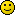» BTW: EMAI is not NTID with low variability but HVD (CVwR = se2CV(swR)).

I know. But I don't have any project with NTID (one of the reasons I waited until now to read the warfarin guidance in detail) so for coding and validating purpose EMA data should be ok.

All the best,
Shuanghe
d_labes
★★★

Berlin, Germany,
2015-09-29 21:55

@ Shuanghe
Posting: # 15495
Views: 18,474

## Another R-solution

Gentlemen (and Ladiesto be politically correct!),

don't praise me to the skies (German: "Über den grünen Klee loben")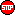.
Not so simple, but also no so complicated. Only tried to re-invent the SAS code in R, one to one.

Regards,

Detlew
gvk
☆

India,
2019-05-21 11:36

@ d_labes
Posting: # 20287
Views: 4,135

## @Shuanghe: Outdated URL

» # Get the data according to Shuanghe» emadata  <- "https://dl.dropboxusercontent.com/u/7685360/permlink/emabe4p.csv"» ema <- read.csv(file = emadata, stringsAsFactors = FALSE)

Dear Detlew,

Can you share the .CSV raw data file used in the program.

M.tareq
☆

2017-04-14 15:04

@ Shuanghe
Posting: # 17239
Views: 13,836

## SAS and R (?) for variability comparison (FDA NTID Guidance)

Dear all,
notation clarification for a newbie,
using EMA's sample data set I -full replicate- after removing the subject that didn't complete all periods
regarding this post : ddubins post
for calculation of CV-intra in full replicate design using FDA SAS code provided in appendix E
FDA Guidance
according to ddubins entry about Covariate Parameter Estimates:
Residual Subject Treatment A 0.124 <---- (sig_WT^2, the within-subject standard deviation) for the Test product Residual Subject Treatment B 0.242 <---- (sig_WR^2, the within-subject standard deviation for the Reference product)
however when using the code given in fda guidance for warfrain sodium and following the same idea here to Calculate the 90% confidence interval of the ratio of the within subject standard
deviation of test product to reference product σWT/σWR by using the residual terms in covariates estiames to calculate the upper bound as follows: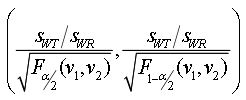guidance for warfrain sodium
and calculating the SWr for the critical bound ,the numbers aren't same ,
Covariance Parameter Estimates Cov Parm Subject Group Estimate FA(1,1) Subject   0.8454 FA(2,1) Subject   0.8232 FA(2,2) Subject   0.02062 Residual Subject Formula R 0.2097 Residual Subject Formula T 0.1202 
and Swr obtained from proc mixed step -same idea as Shuanghe- there's slight differenceCovParm  Estimate s2wr  theta      y       boundy sWR     critbound Residual 0.4080 0.20401 1.11006 -0.22647 -0.17419 0.45168 -0.14657 
my first question is and -sorry for the silly long post,the residual terms in covariates estimates is an estimate of the variance for both Test and reference and not the standard deviation as ddubins said?
and that means the calculation will be as follows :
sqrt(Residual Subject Formula R)
and same for Test to obtain the variability ratio?

2nd question,

Regarding Helmut lecture about Reference Scaled Average Bioequivalence (part II: NTIDs)
RSABE NTIDs
using the data set provided: CNS drug data set
when using the fda code for NTID mentioned above in warfarin guidance i get the following values:
Cov Parm Subject Group Estimate FA(1,1) Subject   0.2473 FA(2,1) Subject   0.2680 FA(2,2) Subject   0 Residual Subject Formula R 0.02483 Residual Subject Formula T 0.003281
and
CVwt=SQRT(EXP(0.003281)-1) --> 5.73% CVwr==SQRT(EXP(0.02483)-1) --> 15.86%
CL: 93.90% - 103.35% CovParm    Estimate   s2wr    theta          y         ]boundy     sWR       critbound Residual   0.03095   0.015474   1.11006   -0.017177   -0.010451   0.12439   -0.009828289
when using these estimates to obtain the upper bound for variability comparison i get : 0.60080159
the number checks out with what's in your lecture regarding EMA values but why there's a difference between FDA values ?Thanks in advance and Best Regards
M.tareq
☆

2017-04-15 01:01

@ M.tareq
Posting: # 17241
Views: 13,727

## SAS and R (?) for variability comparison (FDA NTID Guidance)after watching Jenifer lewrence in passengersi got it, the reason for the different values i got from Helmuts lecture

Thanks, Mis Jenifer and sorry for the long post

but can anyone please explain the difference between the estimates values and the one obtained from the calculation using proc mixedIng. Helmut Schütz